## Default

You can build a dendrogram and heatmap by using the `clustermap()` function of seaborn library. The following example displays a default plot.

``````# Libraries
import seaborn as sns
import pandas as pd
from matplotlib import pyplot as plt

# Data set
url = 'https://raw.githubusercontent.com/holtzy/The-Python-Graph-Gallery/master/static/data/mtcars.csv'
df = df.set_index('model')

# Default plot
sns.clustermap(df)

# Show the graph
plt.show()``````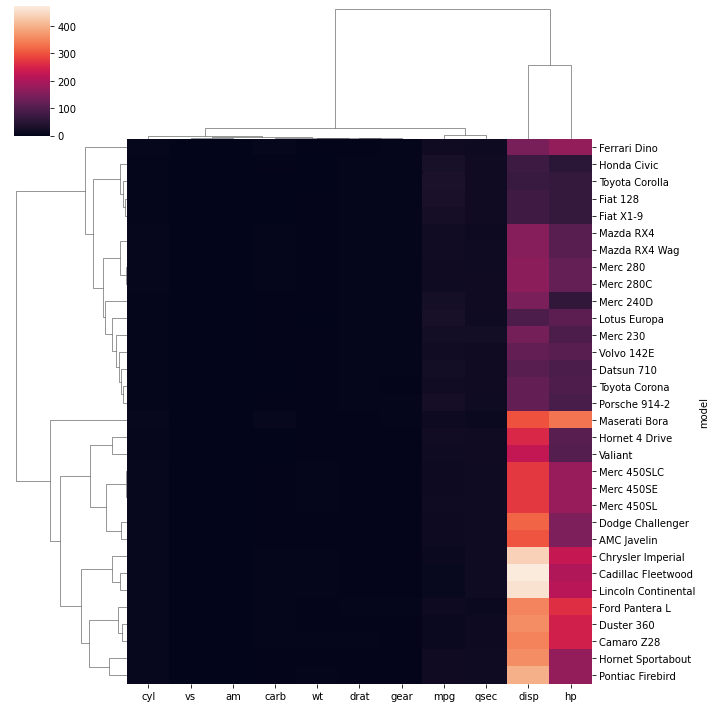## Normalize

It is possible to standardize or normalize the data you want to plot by passing the `standard_scale` or `z_score` aguments to the function:

• `standard_scale` : Either 0 (rows) or 1 (columns)
• `z_score` : Either 0 (rows) or 1 (columns)
``````# Libraries
import seaborn as sns
import pandas as pd
from matplotlib import pyplot as plt

# Data set
url = 'https://raw.githubusercontent.com/holtzy/The-Python-Graph-Gallery/master/static/data/mtcars.csv'
df = df.set_index('model')

# Standardize or Normalize every column in the figure
# Standardize:
sns.clustermap(df, standard_scale=1)
plt.show(
)
# Normalize
sns.clustermap(df, z_score=1)
plt.show()``````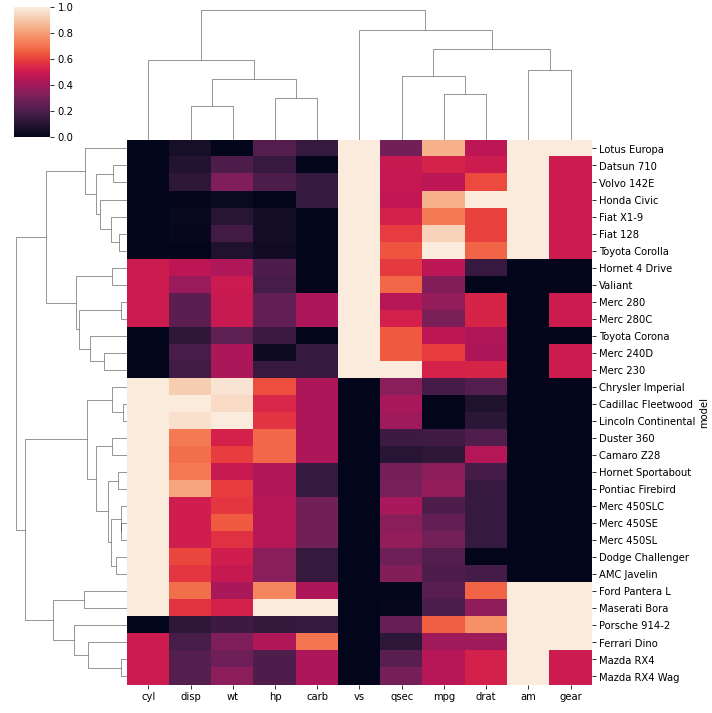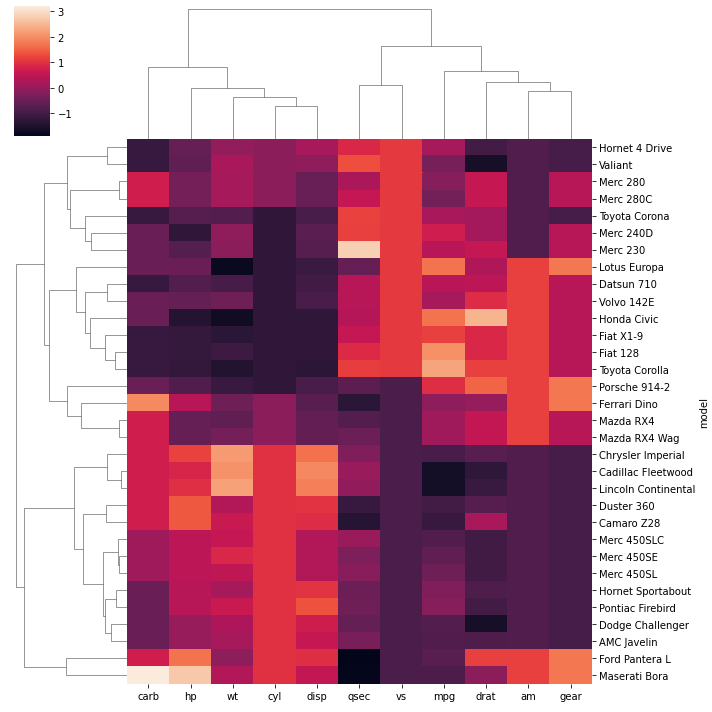## Distance Method

You can use different distance metrics for your data using the `metric` parameter. The most common methods are correlation and euclidean distance.

``````# Libraries
import seaborn as sns
import pandas as pd
from matplotlib import pyplot as plt

# Data set
url = 'https://raw.githubusercontent.com/holtzy/The-Python-Graph-Gallery/master/static/data/mtcars.csv'
df = df.set_index('model')

# plot with correlation distance
sns.clustermap(df, metric="correlation", standard_scale=1)
plt.show()

# plot with euclidean distance
sns.clustermap(df, metric="euclidean", standard_scale=1)
plt.show()``````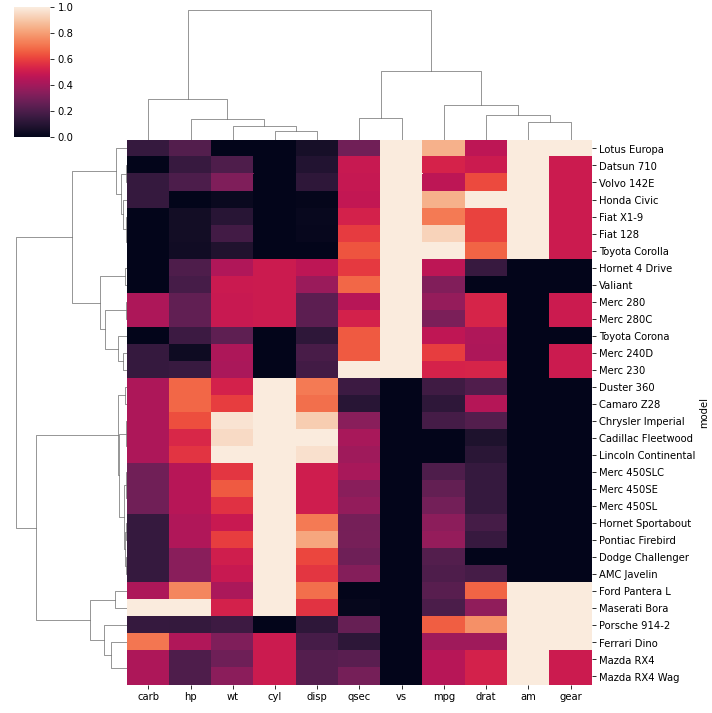## Cluster Method

Since we determined the distance calculation method, now we can set the linkage method to use for calculating clusters with the `method` parameter.

``````# Libraries
import seaborn as sns
import pandas as pd
from matplotlib import pyplot as plt

# Data set
url = 'https://raw.githubusercontent.com/holtzy/The-Python-Graph-Gallery/master/static/data/mtcars.csv'
df = df.set_index('model')

# linkage method to use for calculating clusters: single
sns.clustermap(df, metric="euclidean", standard_scale=1, method="single")
plt.show()

# linkage method to use for calculating clusters: ward
sns.clustermap(df, metric="euclidean", standard_scale=1, method="ward")
plt.show()``````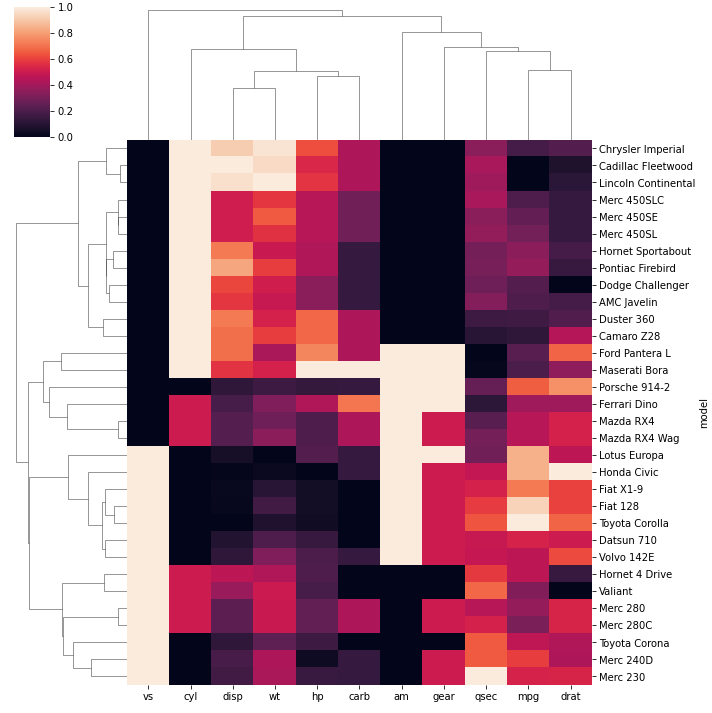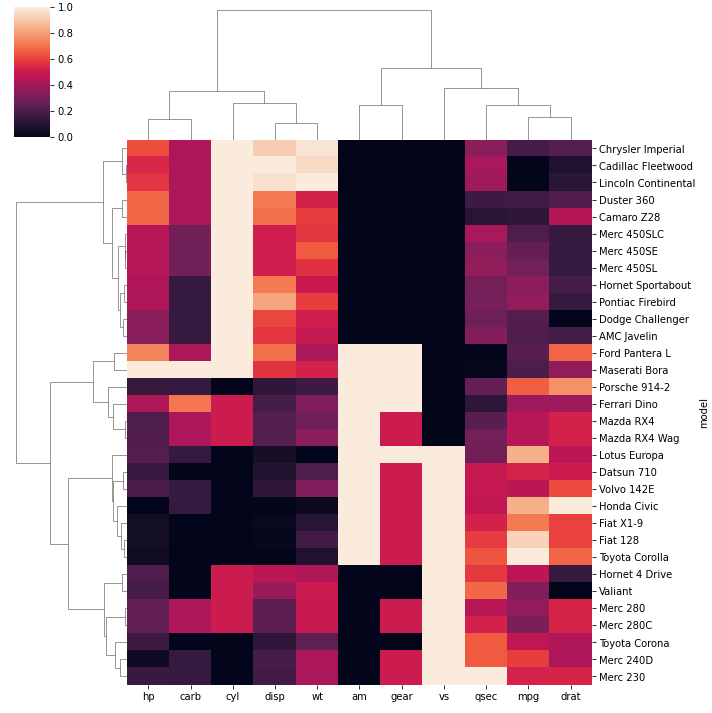## Color

The color palette can be passed to the `clustermap()` function with the `cmap` parameter.

``````# Libraries
import seaborn as sns
import pandas as pd
from matplotlib import pyplot as plt

# Data set
url = 'https://raw.githubusercontent.com/holtzy/The-Python-Graph-Gallery/master/static/data/mtcars.csv'
df = df.set_index('model')

# Change color palette
sns.clustermap(df, metric="euclidean", standard_scale=1, method="ward", cmap="mako")
plt.show()
sns.clustermap(df, metric="euclidean", standard_scale=1, method="ward", cmap="viridis")
plt.show()
sns.clustermap(df, metric="euclidean", standard_scale=1, method="ward", cmap="Blues")
plt.show()``````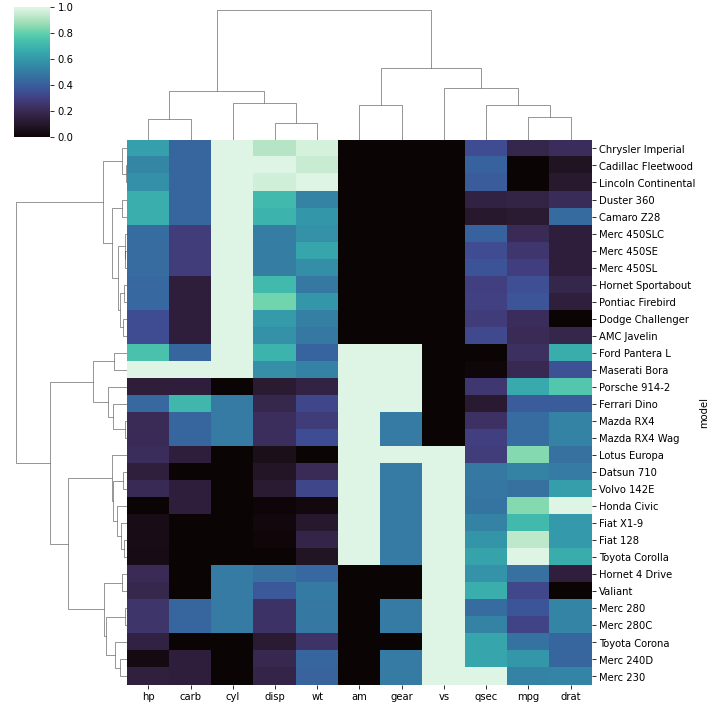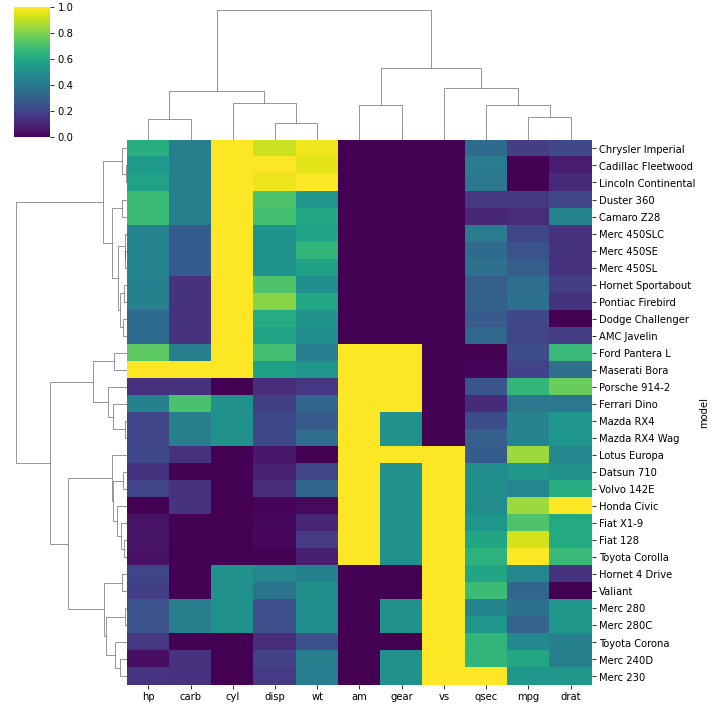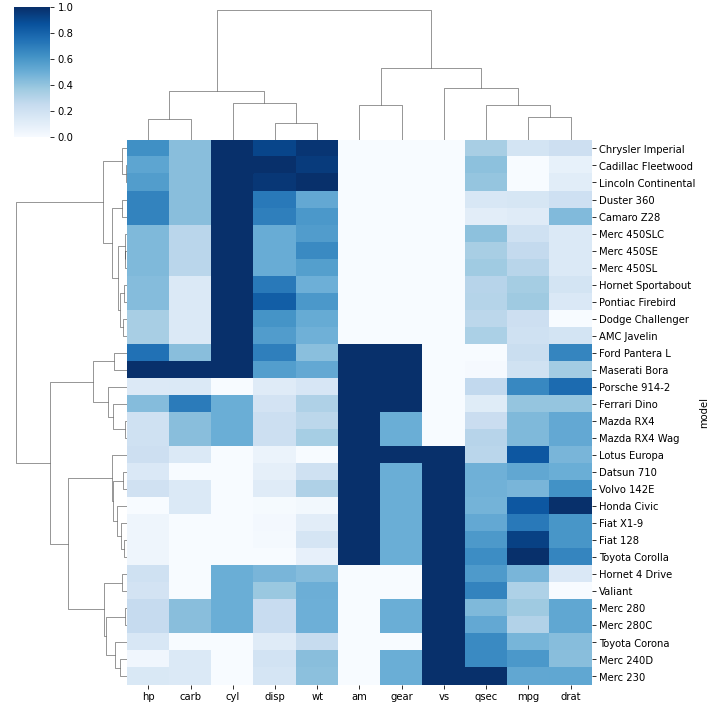## Outliers

In order to ignore an outlier in a heatmap, you can use `robust` parameter:

• `robust` : If True, the colormap range is computed with robust quantiles instead of the extreme values
``````# Libraries
import seaborn as sns
import pandas as pd
from matplotlib import pyplot as plt

# Data set
url = 'https://raw.githubusercontent.com/holtzy/The-Python-Graph-Gallery/master/static/data/mtcars.csv'
df = df.set_index('model')

# Let's create an outlier in the dataset:
df.loc[15:16,'drat'] = 1000

# use the outlier detection
sns.clustermap(df, robust=True)
plt.show()

# do not use it
sns.clustermap(df, robust=False)
plt.show()``````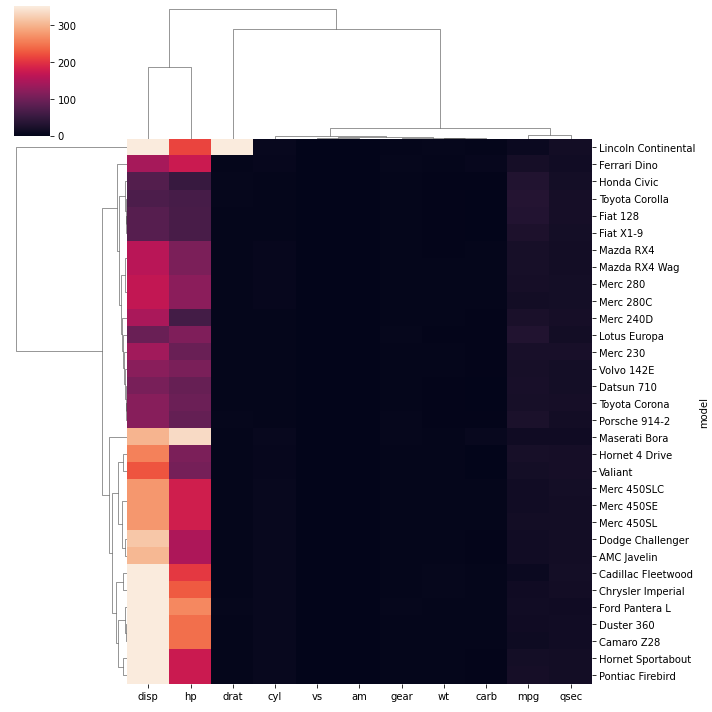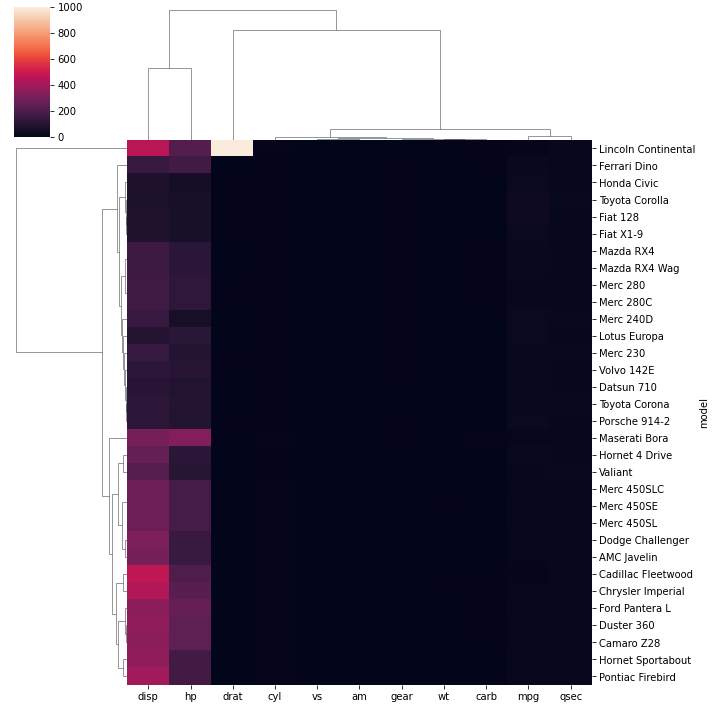## Contact & Edit

👋 This document is a work by Yan Holtz. You can contribute on github, send me a feedback on twitter or subscribe to the newsletter to know when new examples are published! 🔥